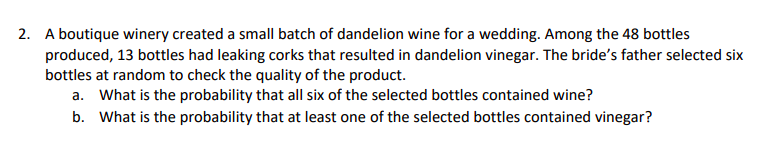# A boutique winery created a small batch of dandelion wine for a wedding. Among the 48 bottlesproduced, 13 bottles had leaking corks that resulted in dandelion vinegar. The bride's father selected sixbottles at random to check the quality of the product.2.What is the probability that all six of the selected bottles contained wine?What is the probability that at least one of the selected bottles contained vinegar?a.b.

Question
12 viewshelp_outlineImage TranscriptioncloseA boutique winery created a small batch of dandelion wine for a wedding. Among the 48 bottles produced, 13 bottles had leaking corks that resulted in dandelion vinegar. The bride's father selected six bottles at random to check the quality of the product. 2. What is the probability that all six of the selected bottles contained wine? What is the probability that at least one of the selected bottles contained vinegar? a. b. fullscreen
check_circle

Step 1

Introduction to binomial distribution:

Consider a random experiment involving n independent trials, such that the outcome of each trial can be classified as either a “success” or a “failure”. The numerical value “1” is assigned to each success and “0” is assigned to each failure.

Moreover, the probability of getting a success in each trial, p, remains a constant for all the n trials. Denote the probability of failure as q. As success and failure are mutually exclusive, q = 1 – p.

Let the random variable X denote the number of successes obtained from the n trials. Thus, X can take any of the values 0,1,2,…,n.

Then, the probability distribution of X is a Binomial distribution with parameters (n, p) and the probability mass function (pmf) of X, that is, of a Binomial random variable, is given as:

Step 2

a.

Obtain the probability that all the six of the selected bottles contained wine:

It is given that the bride’s father has selected 6 bottles randomly to check the quality. That is, the size of the sample taken from the population of bottles produced is n = 6. It is said that it is a random sample, imp-lying independence among each other. As a result, the 6 bottles may be considered as 6 independent trials.

Consider the event of containing a wine as a “success”. It is said that among 48 bottles produced, 13 had leaking corks that resulted in dandelion vinegar. That is, the wine in 13 bottles had turned into vinegar. Now, only 35 bottles has wine among 48 bottles. Thus, the probability that a bottle has wine, that is, the probability of success in each trial is p = 35/48. Then q = 1 – (35/48) = 13/48.

Consider X as the number of bottles that contain wine among the 48 sampled bottles. Then, X has a Binomial distribution with parameters (n = 48, p = 35/48) with pdf as given below:

P(x) = 6Cx*(35/48)x * (13/48)6–x ; x = 0, 1 , 2 , ...,6.

The requirement is all teh 6 bottles should contain wine. That is, X = 6.

The probability that all the six selected bottles contained wine is obtained as 0.1503 from the calculation given below:

Step 3

b.

Obtain the probability that at least one of the selected six bottles contained vinegar:

Consider the event of containing a vinegar as a “success”. It is said that among 48 bottles produced, 13 had leaking corks that resulted in dandelion vinegar. That is, 13 bottles had vinegar among 48 bottles. Thus, the probability that a bottle has vinegar, that is, the probability of success in each trial is p = 13/48. Then q = 1 – (13/48) = 35/48.

Consider Y as the number of bottles that contain vinegar among the 48 sampled bottles. Then, X has a Binomial distrib...

### Want to see the full answer?

See Solution

#### Want to see this answer and more?

Solutions are written by subject experts who are available 24/7. Questions are typically answered within 1 hour.*

See Solution
*Response times may vary by subject and question.
Tagged in

### Other